19 November 2017

# Interconnects

### How to use interconnects

##### Point-based interconnects
```# example created by Bright Photonics

import nazca as nd
import nazca.demofab as demo

demo.shallow.strt(length=50).put(0)
demo.shallow.bend(angle=90).put(0, 50)
demo.shallow.sbend(offset=20).put(0, 100)
demo.shallow.taper(length=50, width1=2, width2=10).put(0, 150)
demo.shallow.ptaper(length=50, width1=2, width2=10).put(0, 200)

nd.export_gds()```

An example with different point-based interconnects: straight waveguide (strt), bend waveguide (bend), sbend, taper and parabolic taper. Use demofab_klayout_colors.lyp to view the mask in KLayout.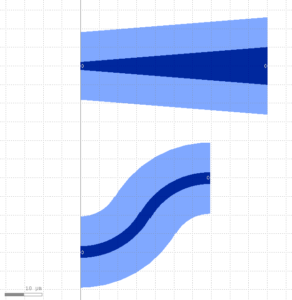##### Point-to-point (p2p) based interconnects
```# example created by Bright Photonics

import nazca as nd
import nazca.demofab as demo

s1 = demo.shallow.strt(10).put(0)
s2 = demo.shallow.strt(30).put(100, 40, 120)
demo.shallow.strt_bend_strt_p2p(pin1=s1, pin2=s2.pin['a0']).put()

nd.export_gds()```

An example of straight-bend-straight point-to-point interconnect. Use demofab_klayout_colors.lyp to view the mask in KLayout.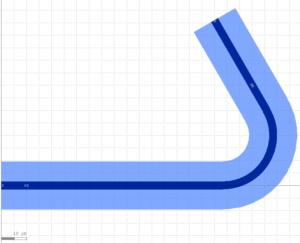```# example created by Bright Photonics

import nazca as nd
import nazca.demofab as demo

s1 = demo.shallow.strt(10).put(0)
s2 = demo.shallow.strt(30).put(100, 40, 120)
demo.shallow.bend_strt_bend_p2p(pin1=s1, pin2=s2.pin['a0']).put()

nd.export_gds()```

An example of bend-straight-bend point-to-point interconnect. Use demofab_klayout_colors.lyp to view the mask in KLayout.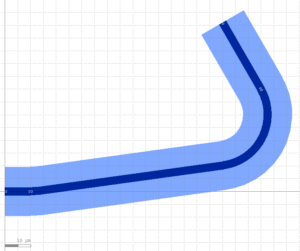```# example created by Bright Photonics

import nazca as nd
import nazca.demofab as demo

s1 = demo.shallow.strt(10).put(0)
s2 = demo.shallow.strt(30).put(100, 40, 0)
demo.shallow.sbend_p2p(pin1=s1, pin2=s2.pin['a0']).put()

nd.export_gds()```

An example of sbend point-to-point interconnect. Use demofab_klayout_colors.lyp to view the mask in KLayout.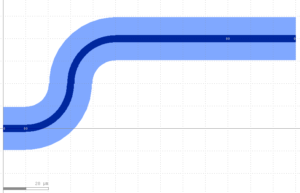```# example created by Bright Photonics

import nazca as nd
import nazca.demofab as demo

s1 = demo.shallow.strt(10).put(0)
s2 = demo.shallow.strt(30).put(100, 40, 0)
demo.shallow.ubend_p2p(pin1=s1, pin2=s2).put()

nd.export_gds()```

An example of ubend point-to-point interconnect. Use demofab_klayout_colors.lyp to view the mask in KLayout.```# example created by Bright Photonics

import nazca as nd
import nazca.demofab as demo

s1 = demo.shallow.strt(10).put(0)
s2 = demo.shallow.strt(30).put(100, 40, 120)
demo.shallow.strt_p2p(pin1=s1, pin2=s2.pin['a0']).put()

nd.export_gds()```

An example of straight point-to-point waveguide. Use demofab_klayout_colors.lyp to view the mask in KLayout.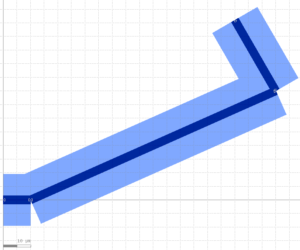## Related Tutorials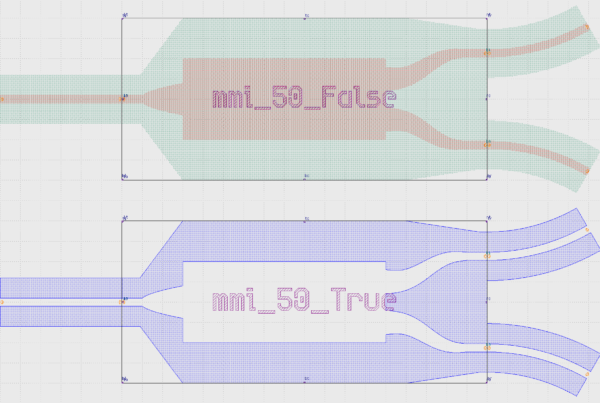In this example we show how to create an inverted MMI with custom interconnects.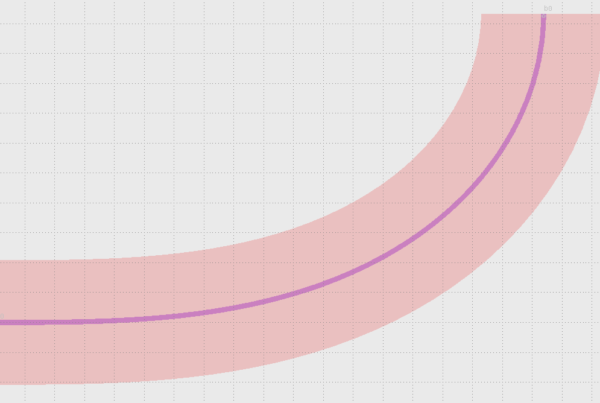In this example we show how to use Euler bends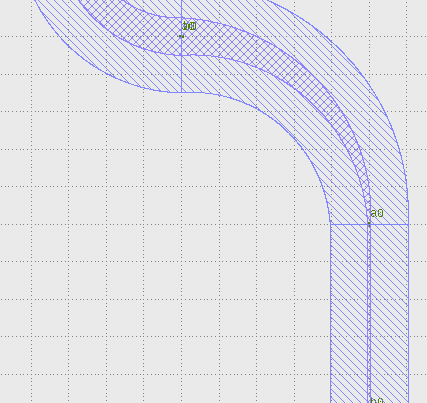In this example we show how to create a free form parametric curve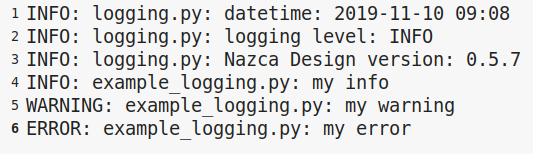In this example we show how to log your layout.